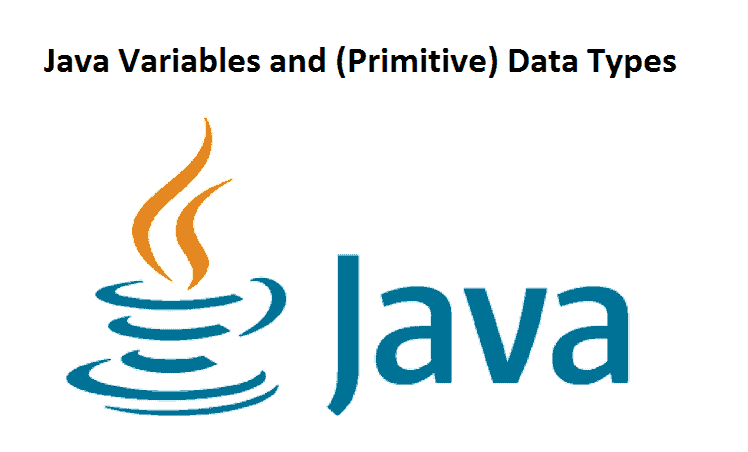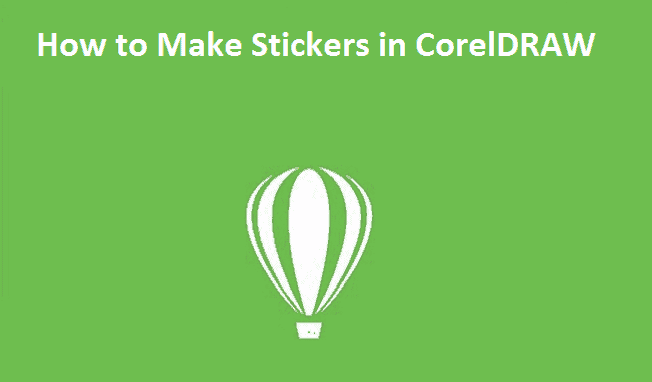# Java Data Types (Primitive)Java Variables and (Primitive) Data Types

In this tutorial, we will learn about pretty much every one of the 8 primitive data types in Java with the help of examples.

Contents

## Java Data Types

As the name proposes, data types in Java indicate the type of data that can be stored inside Java variables.

Java is a statically-typed language. This implies that all variables should be announced before they can be used.

``int speed;``

Here, speed is a variable, and the data type of the variable is int.

The int data type determines that the speed variable can only contain integers.

There are 8 data types predefined in Java programming language, known as primitive data types.

Note: In addition to primitive data types, there are also referenced types (object type).

## 8 Primitive Data Types

### 1. boolean type

• The boolean data type has two possible values, either true or false.
• Default value: false.
• They are usually used for true/false conditions. For example,

#### Example 1: Java boolean data type

``````class Main {
public static void main(String[] args) {

boolean flag = true;
System.out.println(flag);    // prints true
}
}``````

## 2. byte type

• The byte data type can have values from –128 to 127 (8-bit signed two’s complement integer).
• If it’s certain that the value of a variable will be within -128 to 127, then it is used instead of int to save memory.
• Default value: 0

### Example: 2: Java byte data type

``````class Main {
public static void main(String[] args) {

byte range;
range = 124;
System.out.println(range);    // prints 124
}
}``````

## 3. short type

• The short data type can have values from –32768 to 32767 (16-bit signed two’s complement integer).
• If it’s certain that the value of a variable will be within -32768 and 32767, then it is used instead of other integer data types (int, long).
• Default value: 0

### Example 3: Java short data type

``````class Main {
public static void main(String[] args) {

short temperature;
temperature = -200;
System.out.println(temperature);  // prints -200
}
}``````

## 4. int type

The int data type can have values from -231 to 231-1 (32-bit signed two’s complement integer).
If you are using Java 8 or later, you can use an unsigned 32-bit integer with a minimum value of 0 and a maximum value of 232-1. If you are interested in learning more about it, visit: How to use the unsigned integer in java 8?
Default value: 0

### Example 4: Java int data type

``````class Main {
public static void main(String[] args) {

int range = -4250000;
System.out.println(range);  // print -4250000
}
}``````

## 5. long type

• The long data type can have values from 263 to 263 -1 (64-bit signed two’s complement integer).
• If you are using Java 8 or later, you can use an unsigned 64-bit integer with a minimum value of 0 and a maximum value of 264-1.
• Default value: 0

### Example 5: Java long data type

``````class LongExample {
public static void main(String[] args) {

long range = -42332200000L;
System.out.println(range);    // prints -42332200000
}
}``````

Notice, the use of L toward the finish of – 42332200000. This speaks to that it’s a vital exacting of the long type. You will find out about integral literals later in this article.

## 6. double type

• The double data type is a double-precision 64-bit floating-point.
• It should never be used for precise values such as currency.
• Default value: 0.0 (0.0d)

## Example 6: Java double data type

``````class Main {
public static void main(String[] args) {

double number = -42.3;
System.out.println(number);  // prints -42.3
}
}``````

## 7. float type

The float data type is a single-precision 32-bit floating-point. Learn more about single-precision and double-precision floating-point if you are interested.
It should never be used for precise values such as currency.
Default value: 0.0 (0.0f)

### Example 7: Java float data type

``````class Main {
public static void main(String[] args) {

float number = -42.3f;
System.out.println(number);  // prints -42.3
}
}``````

Notice that, we have used -42.3f instead of -42.3in the above program. It’s because -42.3 is a double literal. To tell the compiler to treat -42.3 as float rather than double, you need to use f or F.

If you want to know about single-precision and double-precision, visit Java single-precision and double-precision floating-point.

## 8. char type

• It’s a 16-bit Unicode character.
• The minimum value of the char data type is ‘\u0000’ (0). The maximum value of the char data type is ‘\uffff’.
• Default value: ‘\u0000’

### Example 8: Java char data type

``````class Main {
public static void main(String[] args) {

char letter = '\u0051';
System.out.println(letter);  // prints Q
}
}``````

Here, the Unicode value of Q is \u0051. Hence, we get Q as the output.

Here is another example:

``````class Main {
public static void main(String[] args) {

char letter1 = '9';
System.out.println(letter1);  // prints 9

char letter2 = 65;
System.out.println(letter2);  // prints A

}
}``````

Here, we have assigned 9 as a character (determined by single statements) to the letter1 variable. However, the letter2 variable is allocated 65 as a number (no single statements).

Subsequently, A is printed to the output. It is on the grounds that Java regards characters as vital sorts and the ASCII value of An is 65. To learn more about ASCII, visit What is ASCII Code?

## String type

Java additionally offers support for character strings by means of java. lang.String class. Strings in Java are not primitive sorts. All things considered, they are objects. For instance,

``String myString = "Java Programming";``

Here, myString is an object of the String class. To learn more, visit Java Strings.

Thanks for reading! We hope you found this tutorial helpful and we would love to hear your feedback in the Comments section below. And show us what you’ve learned by sharing your photos and creative projects with us.### How to Make a Window Sticker in CorelDRAW### How to Make Stickers in CorelDRAW